Process是spss中处理中介和调节模型的插件, 我们今天要讲一下process中的model1, 也就是最简单的调节模型, 视频教程已经放在文章末尾。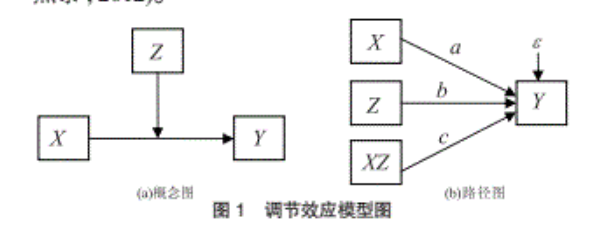• 将自变量和调节变量进行中心化
• 计算自变量和调节变量的乘积
• 使用回归检验一下方程中系数c的显著性:

$$Y = i + aX + bZ + cXZ + e$$

• 1.为什么要中心化?
• 2.为什么乘积项的系数显著就可以证明存在调节效应?

### 为什么要中心化

$$Y = i + aX + e$$

$$Y = i + (a+\bar Z)X + b \bar Z + e ; (\bar Z ≠ 0)$$

### 为什么乘积项的系数显著就可以证明存在调节效应

c≠0显著时, 方程如下, 实际上a+cZ就是以X为自变量, 以Y为因变量的回归方程的斜率, c不为0, 说明斜率会因为Z的改变而改变:

$$Y = i + (a+cZ)X + bZ + e$$

c=0时, 方程如下, 也就是说Z的变化只引起了方程的截距的改变, 但是并没有影响方程的斜率:

$$Y = i + aX + bZ + e$$

### SPSS分析过程

• 计算自变量和调节变量的均值和标准差
• 中心化
• 求乘积
• 做两个回归模型

#### 计算自变量和调节变量的均值和标准差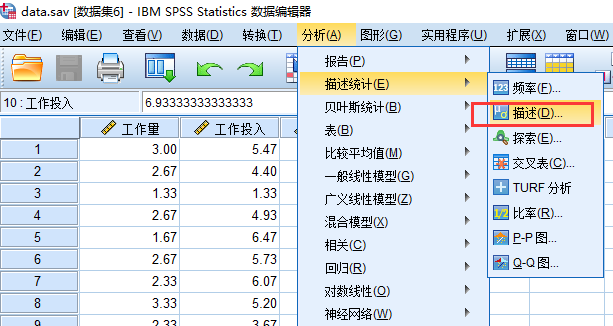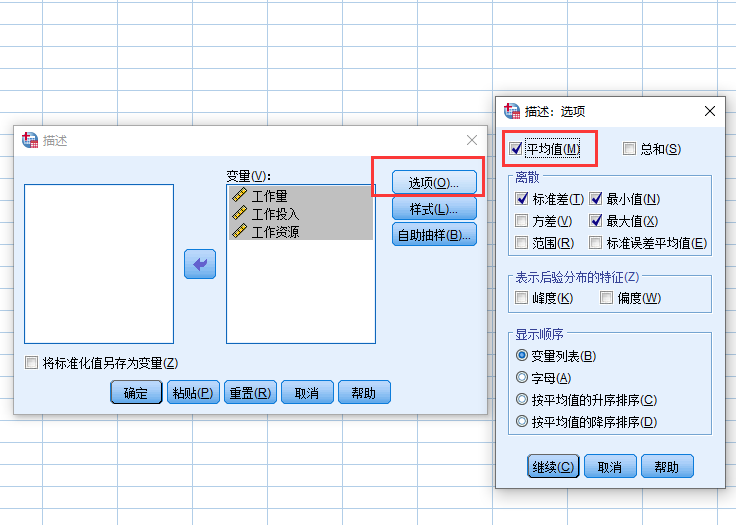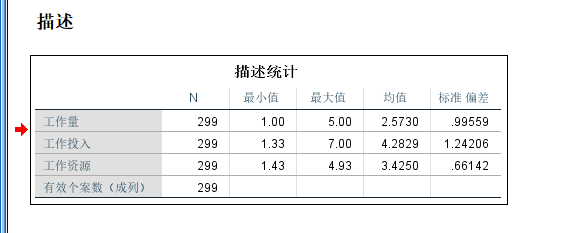#### 中心化变量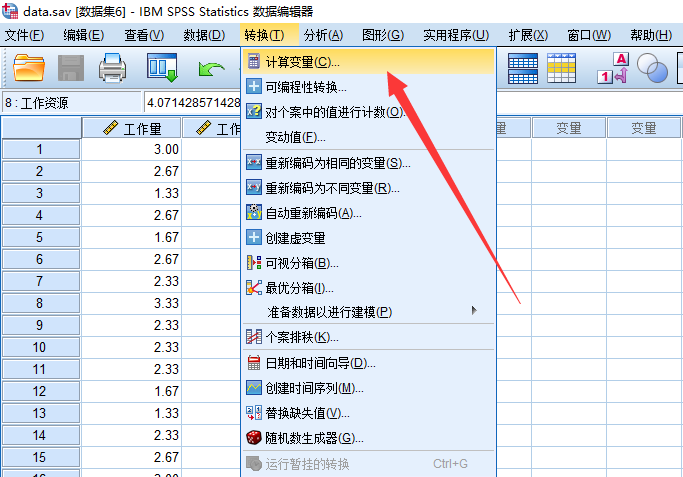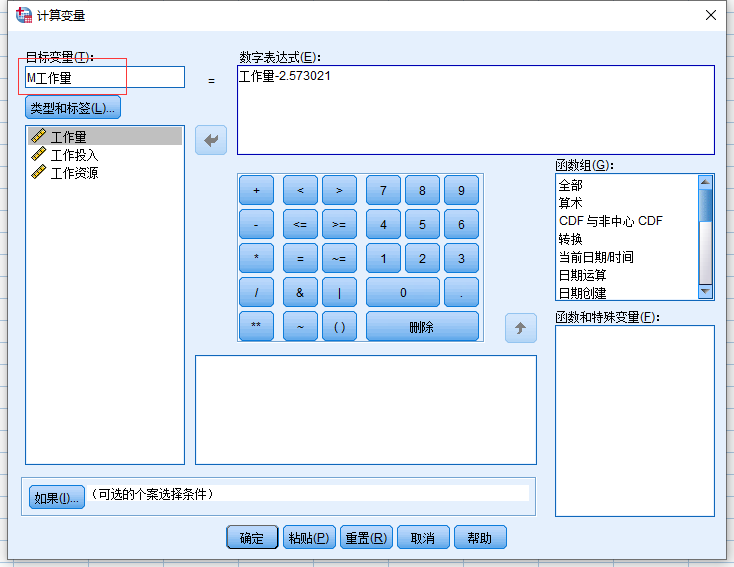#### 计算交互项(乘积)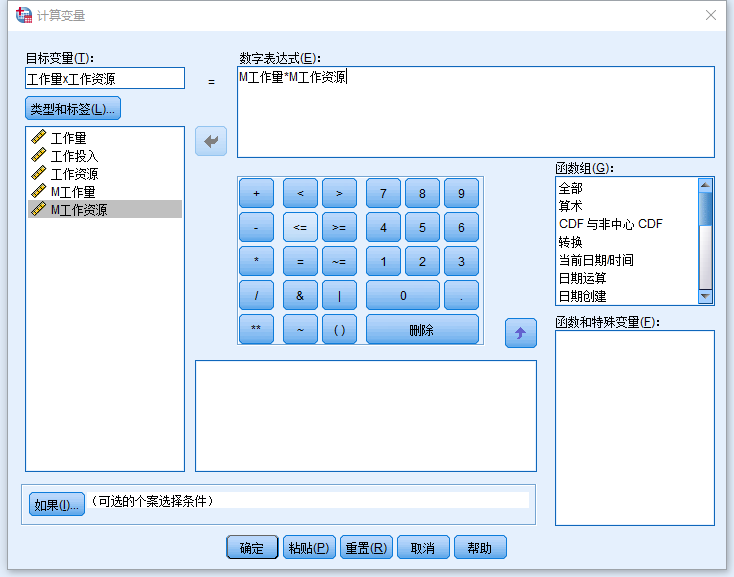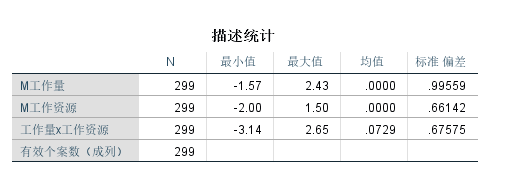#### 做回归

$$𝑌=𝑖+𝑎𝑋+𝑏𝑍+𝑒 𝑌=𝑖+𝑎𝑋+𝑏𝑍+𝑐𝑋𝑍+𝑒$$

Step1: 菜单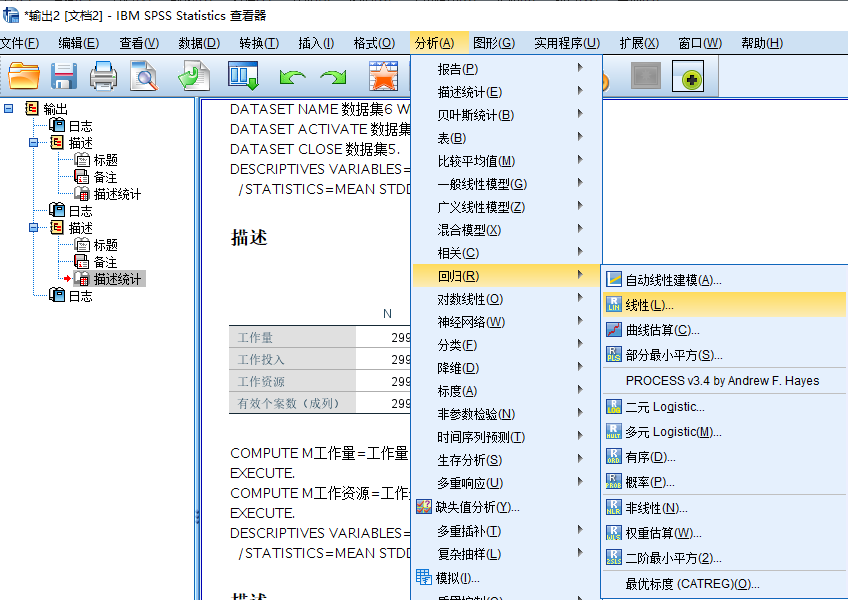Step2:第一个回归模型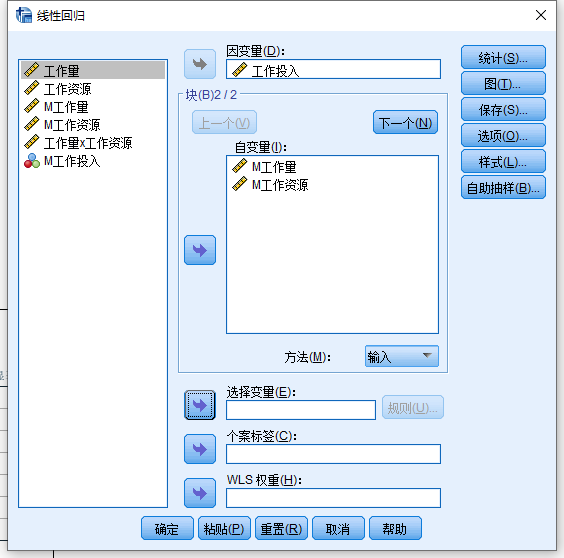Step3:第二个回归模型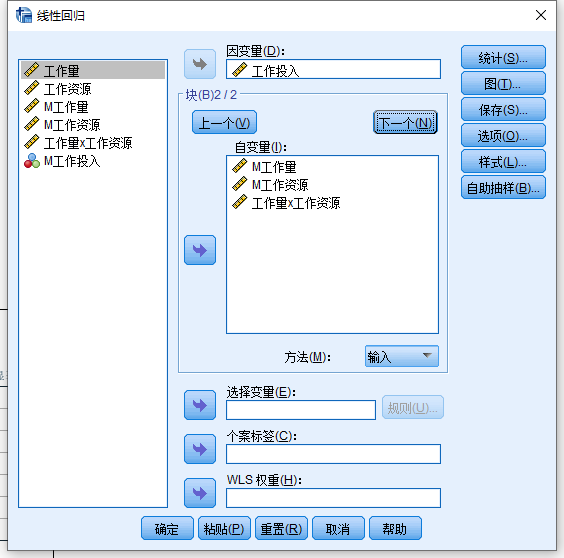Step4:配置选项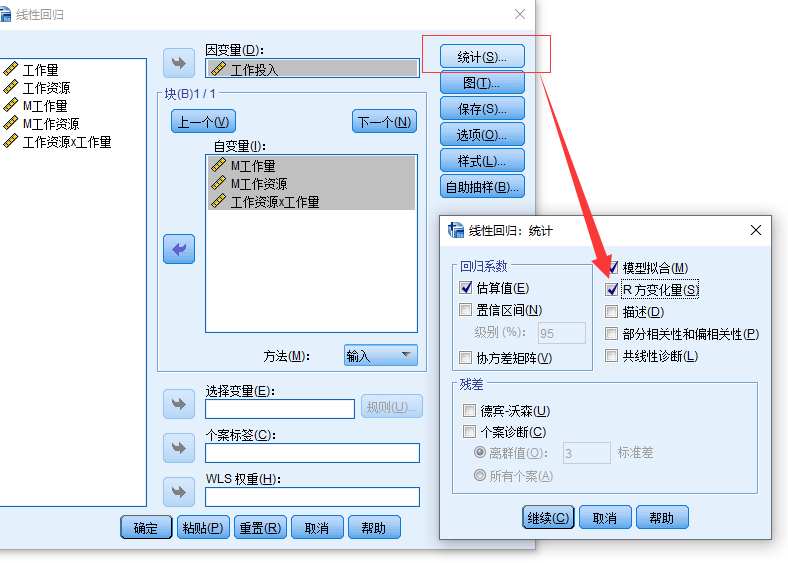Step4:输出结果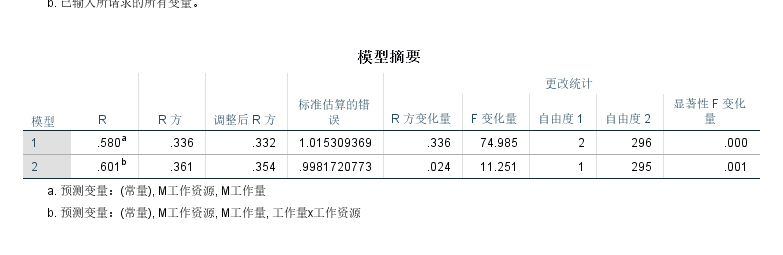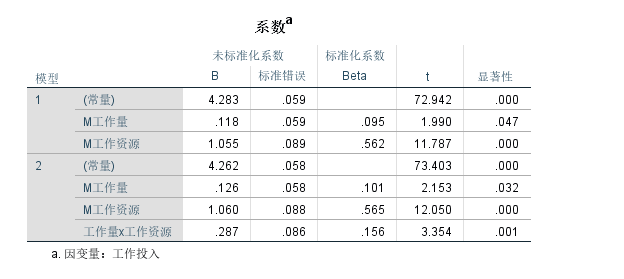### Process做简单交互效应

Process能帮助你更快的检验交互效应, 因为它自动化处理了上面的过程, 下面我们来演示一下如何使用Process插件:

#### 操作方法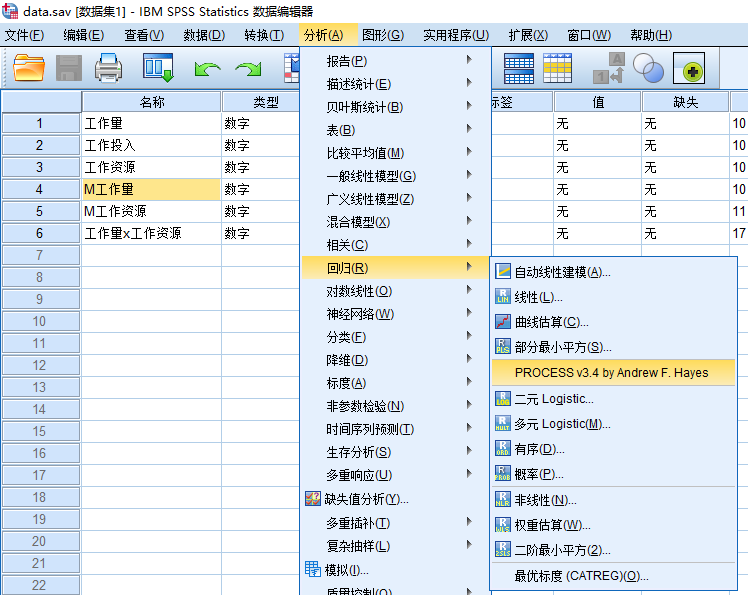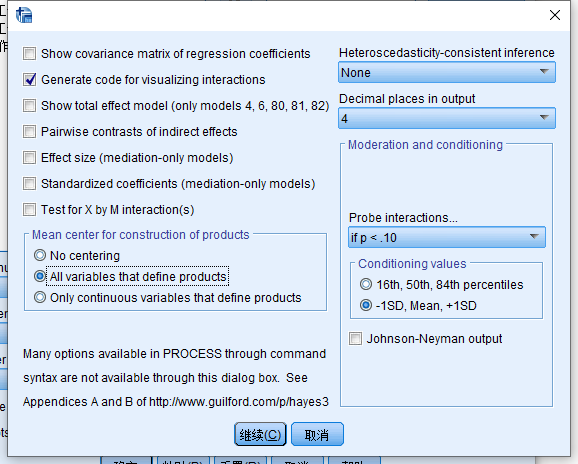• Generate code for visualizing interactions(生成可视化交互效应的代码)
• all variables that define products(变量中心化处理)#### 结果解读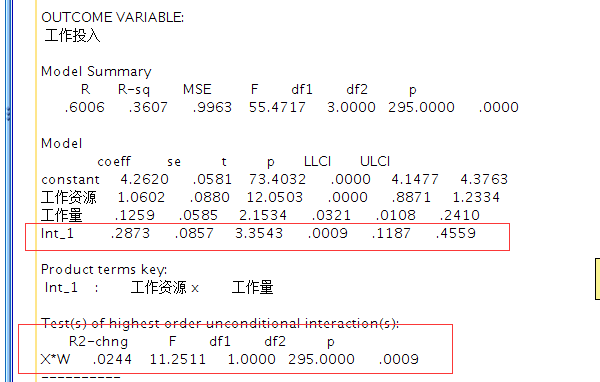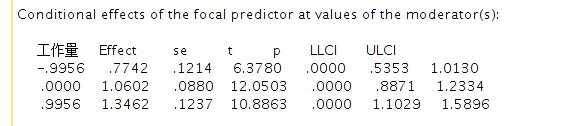#### 结果可视化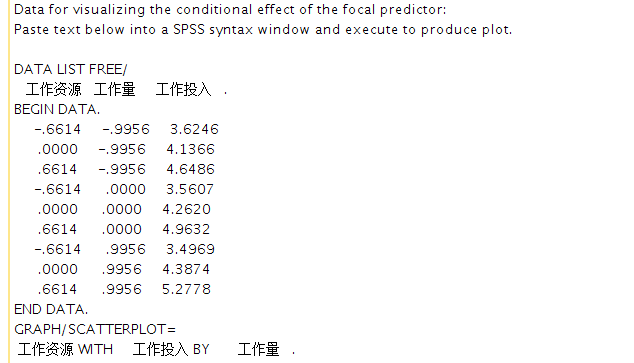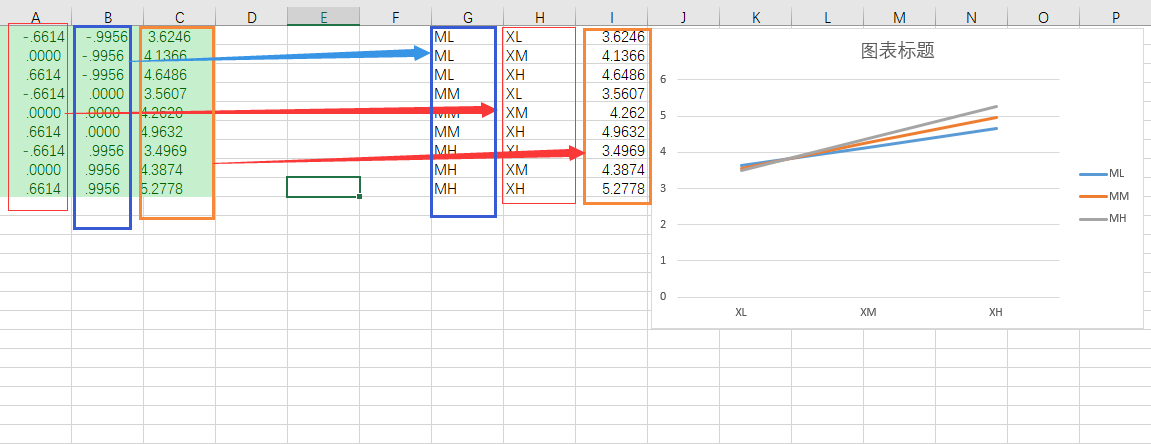### 可视化工具

$$𝑌=𝑖+𝑎𝑋+𝑏𝑍+𝑐𝑋𝑍+𝑒$$

i =

a =

b =

c =

### 总结

• 我们这篇文章介绍了两种方法来检验调节效应, 两种方法本质上都是做了两次回归, 结果也是一样的。
• Process的好处是大大简化了我们的操作步骤

### 赞助